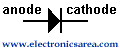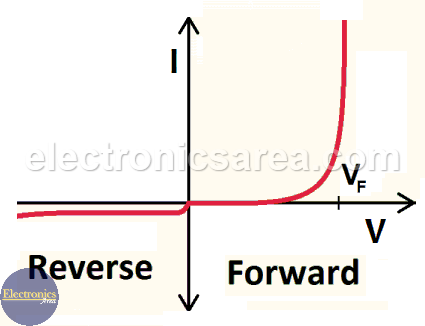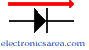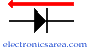# The Semiconductor Diode

The semiconductor diode is the simplest semiconductor device and can be found in almost any electronic circuit. Diodes are manufactured with germanium and silicon (the most common). Diodes are composed of two parts, the “N-layer” (cathode) and the “P-layer” (anode) , which are separated by a barrier.

This barrier has 0.3 volts in the germanium diode and approximately 0.6 volts in the silicon diode.## Principle of operation of a diode

The diode N-layer has free electrons and the diode P-layer has free holes (absence of electrons).

– When a positive voltage is applied to the P-layer and a negative voltage to the N-layer, the electrons in the N layer are pushed to the P layer and electrons flow through the P material beyong the limits of the semiconductor.

Likewise the holes in the P material are pushed, with a negative voltage, to the N material side. Then the holes run through the N material.

– When a positive voltage is applied to the N-layer and a negative to the P-layer, the electrons in the P-layer are pushed to the N-layer and the holes of the N layer are pushed to the P layer. In this case the electrons in the semiconductor don’t move and the electric current doesn´t flow.

## Real diode Current-Voltage RelationshipVF is 0.3 volts in the germanium diode and approximately 0.6 volts in the silicon diode.

Semiconductor diode work in 2 different ways:

## Forward biasedIn this case the electric current circulates through the diode, following the path of the arrow (the arrow of the diode), or from anode to cathode. The electric current passes through out the diode very easily behaving almost like a short circuit.

## Reverse biasedIn this case the electric current in the diode wants to circulate on opposite direction of the arrow (the arrow of the diode), or from the cathode to the anode. The electric current doesn’t flow through the diode, and it behaves as an open circuit.

Note: The operation mentioned before refers to the ideal diode, meaning that we take the diode like a perfect component.

## Semiconductor diode applications

Diodes have many applications, but the most common one is the process of conversion from alternating current (A.C.) to continuous current (C.C.). In this case the diode is used like a rectifier diode.

Other diode applications:

• Reverse voltage protection
• Flyback diode in inductive loads
• etc.
• 2
•
•
•
•
•
•
•
•
2
Shares
•
2
Shares
• 2
•
•
•
•
•
•
•